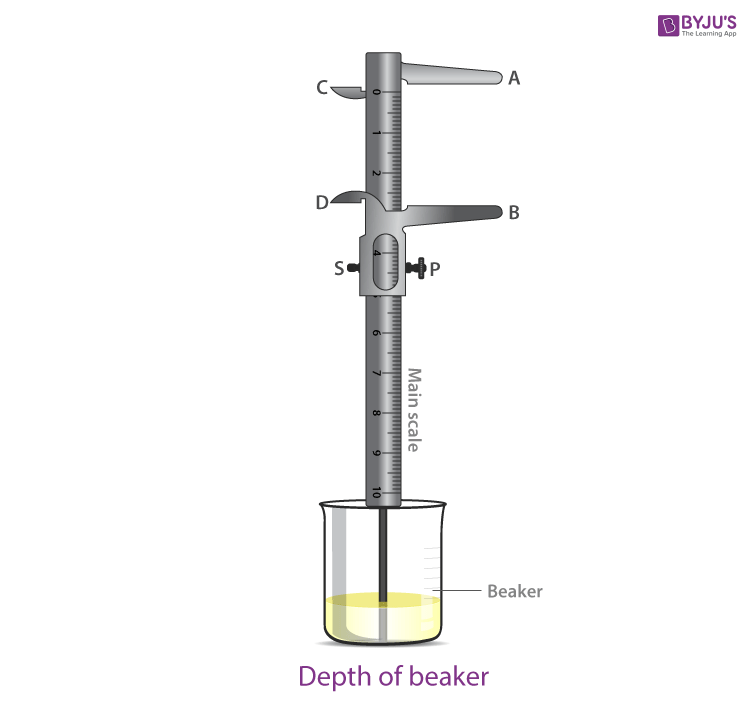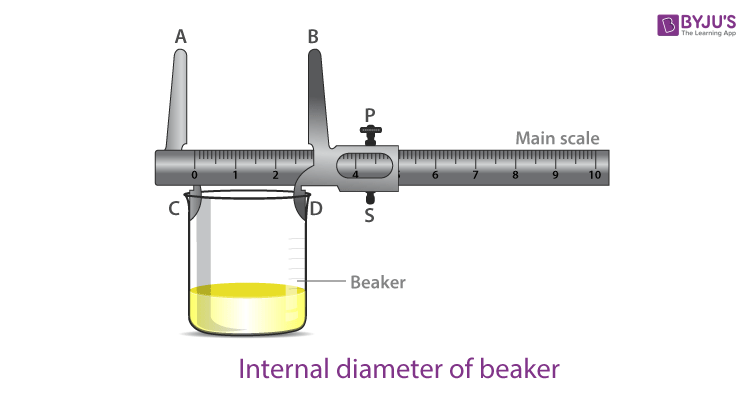# To Measure Internal Diameter and Depth of a Given Beaker/Calorimeter Using Vernier Calipers and Hence Find Its Volume

Vernier calliper is a measuring device with two scales: the main scale and the vernier scale, such that the vernier scale slides over the main scale. The main scale is divided into small divisions in cm and mm and has two fixed jaws A and C. B and D are the jaws of the vernier scale. When the jaws of scales are made to touch, the zeros of both scales coincide. Below is an experiment to determine a beaker’s internal diameter and depth and find the volume.

## Aim

To measure the internal diameter and depth of a beaker/calorimeter using vernier callipers and find its volume.

## Materials Required

1. Vernier calipers
2. A cylindrical object like a beaker
3. Magnifying glass

## Theory

### What Is Least Count?

The least count is defined as the smallest change in the measured quantity, which can be resolved on an instrument’s scale.

### How to Calculate Least Count?

The least count of vernier caliper = Least count of vernier caliper =

$$\begin{array}{l}\frac{frac\,magnitude\,of\, the\, smallest\, division\, on \,the \,main \,scale}{total\, number\, of\, small \,divisions\, on \,the \,vernier\, scale}\end{array}$$

The formula used to calculate the volume of beaker/calorimeter = internal area of cross-section × depth

 $$\begin{array}{l}V=(\frac{D}{2})^{2}.d\end{array}$$

Where,

• D is the internal diameter of the beaker/calorimeter
• d is the depth of the beaker/calorimeter

## Diagram## Procedure

1. Determine and record the least count of the vernier calliper, also known as the vernier constant.
2. To find the zero error, bring the movable jaw BD in contact with the fixed jaw AC. Repeat and record this three times. If there is no zero error, then record zero error as nil.
3. Now put the jaws C and D inside the beaker and open them till they make contact with the inner wall of the beaker without any pressure. Tighten the screw without putting too much pressure.
4. On the main scale, record the zero mark of the vernier scale. Just before the zero mark of the vernier scale, record the main scale reading, which is known as main scale reading (M.S.R).
5. Let n be the number of the vernier scale division, which coincides with the main scale division.
6. Rotate the vernier calliper at 90° and repeat steps 4 and 5 to measure the internal diameter in a perpendicular direction.
7. To measure the depth, find the total reading and zero correction.
8. The edge of the main scale of the vernier calliper should be placed on the peripheral edge. Care should be taken to make the strip go freely inside the beaker and its depth.
9. Once the moving jaw of the vernier calliper touches the bottom of the beaker perpendicularly, the screw of the vernier calliper should be tightened.
10. For four different positions along the circumference of the beaker, repeat steps 4 and 5.
11. Find the total reading and also zero correction.
12. For internal diameter, take two different mean values and for depth, take four different values.
13. Calculate the volume using the proper formula and record the same in the result with units.

## Observations

1. Determination of the least count of vernier caliper1 M.S.D = 1 mm 10 V.S.D = 9 M.S.D

∴ 1 V.S.D = 9/10 M.S.D = 0.9 mm

The least count of vernier caliper (V.C) = 1 M.S.D – 1 V.S.D = (1-0.9) mm = 0.1 mm = 0.01 cm

2. Zero error = (i)…. cm (ii)…. cm (iii)…..cm Mean zero error (e) = …..cm

Mean zero correction © = -(e) = …….cm

3. Table for internal diameter (D)
 Sl. no Main scale Vernier scale reading Total reading No.of vernier division coinciding (n) Value [n×(V.C)] Observed D0=N+n×V.C Corrected D=D0+c 1. D1= 2. D2= 3. D3= 4. D4=
4. Table for the depth (d)
 Sl.no Position Main scale reading (N in cm) Vernier scale reading Total reading No.of vernier division coinciding (n) Value [n×(V.C)] Observed d0=N+n × (V.C) Corrected d=d0+c 1. at A d1= 2. at B d2= 3. at C d3= 4. at D d4=

## Calculations

Mean corrected internal diameter,

$$\begin{array}{l}D=\frac{D_{1}(a)+D_{1}(b)}{2}=…..\;cm\end{array}$$

Mean corrected depth,

$$\begin{array}{l}d=\frac{d_{1}+d_{2}+d_{3}+d_{4}}{4}=…..\;cm\end{array}$$

The volume of the beaker,

$$\begin{array}{l}V=\pi (\frac{D}{2})^{2}d=….\;cm^{3}\end{array}$$

## Result

The volume of the beaker is …….cm3.

## Precautions

1. Apply machine oil or grease to make the vernier scale slide smoothly over the main scale.
2. Do not exert more pressure on the vernier screw to avoid damage to threads.
3. To avoid errors due to parallax, keep the eye directly over the division mark.
4. The significant figures and units used in observations must be correct.

## Sources of Error

1. Not accounting for the zero error in the instrument.
2. Avoid gaps and undue pressure with respect to the placing of vernier callipers.

## Viva Questions

Q1. What is the principle behind the vernier scale?

Ans: The principle behind the vernier scale is that the number of vernier scale divisions coinciding with the main scale division should be either one less or one more.

 $$\begin{array}{l}1 V.S.D=\frac{1 M.S.D}{no.\;of\;divisions\;on\;V.S}\end{array}$$

Q2. What is vernier constant?

Ans: Vernier constant is defined as the difference between the value of one main scale division and one vernier scale division on the vernier callipers.

Q3. What is the least count of a measuring instrument?

Ans: The least count of a measuring instrument is defined as the least quantity that can be measured accurately.

Q4. What is the least count of the vernier calliper?

Ans: The least count of the vernier caliper is 0.1 mm.

Q5. What is zero error?

Ans: Zero error is defined as how far away an instrument’s reading is from zero. It can be either positive or negative.